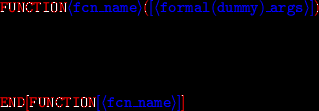Next: Actual arguments, formal (dummy) Up: The Building Blocks of Previous: External Procedures   Contents

# External Functions

The syntax of a function declaration is

For example, suppose we want to declare a function that receives the three coordinates of a cartesion vector x,y,z and returns the euclidian norm of the vector,(this is also called the 2-norm). The arguments x,y,z are REAL, and so is the returned value r.

The declaration could then be

! function that computes norm 2    real=type of the returned value
real function norm_2(x,y,z) !x,y,z are "dummy" arguments
implicit none !scope=body of the function
real::x,y,z !dummy args declaration
norm_2=SQRT(x**2+y**2+z**2)
!function name behaves like a variable
!holding the return value
end function norm_2

!function for norm 1
real function norm_1(x,y,z)
implicit none
real::x,y,z
norm_1=abs(x)+abs(y)+abs(z)
end function norm_1

!function for norm infinity
real function norm_inf(x,y,z)
implicit none
real::x,y,z
norm_inf=max(abs(x),abs(y),abs(z))
end function norm_inf

!the main program
program norms
implicit none
real::a,b,c
real,external::norm_1,norm_2,norm_inf
print*,'The 2-norm= ',norm_2(a,b,c) !a,b,c are "actual" args
print*,'The 1-norm= ',norm_1(a,b,c)
print*,'The inf-norm= ',norm_inf(a,b,c)
end program norms


Note that the function name (norm_2) behaves like a variable; when the function terminates, this variable holds the result of the function (the return value'' of the function). The type of function is the type of the returned result, i.e. the type of the result variable (norm2). This type is declared explicitly as a prefix to the function name; in our example,

real function norm2(x,y,z).

Alternatively, this declaration can be mixed with the other declarations, for example
function norm2(x,y,z)
implicit none
real :: x, y, z, norm2

Either form is valid, and one declaration should always be given (otherwise the compiler will signal an error).

The variables x,y,z are called formal (dummy) arguments. They hold the input data for the function. When the function is invoked, they will be replaced by actual values.

The calling program also declares the type of the function, padded with the EXTERNAL attribute. For example, the calling program might read in the coordinates and print the 2-norm of the vector:

program print_norm
implicit none
real, external :: norm2
real :: a, b, c
print*, 'input~3~coordinates:'